## Matrix Representation of Geometric Transformations

You can use a geometric transformation matrix to perform a global transformation of an image. First, define a transformation matrix and use it to create a geometric transformation object. Then, apply a global transformation to an image by calling `imwarp` with the geometric transformation object. For an example, see Perform Simple 2-D Translation Transformation.

### 2-D Affine Transformations

The table lists 2-D affine transformations with the transformation matrix used to define them. For 2-D affine transformations, the last column must contain [0 0 1] homogeneous coordinates.

Use any combination of 2-D transformation matrices to create an `affine2d` geometric transformation object. Use combinations of 2-D translation and rotation matrices to create a `rigid2d` geometric transformation object.

2-D Affine TransformationExample (Original and Transformed Image)Transformation Matrix
Translation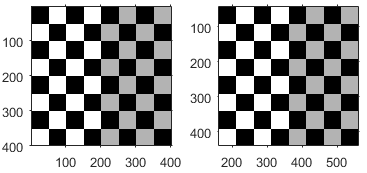`t`x specifies the displacement along the x axis

`t`y specifies the displacement along the y axis.

Scale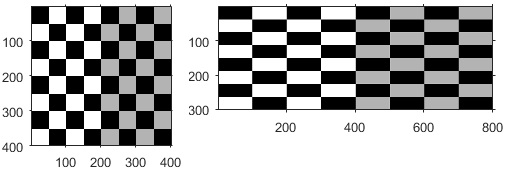`s`x specifies the scale factor along the x axis

`s`y specifies the scale factor along the y axis.

Shear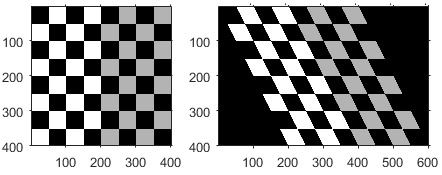`sh`x specifies the shear factor along the x axis

`sh`y specifies the shear factor along the y axis.

Rotation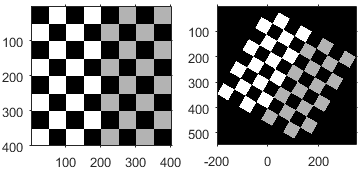`q` specifies the angle of rotation about the origin.

### 2-D Projective Transformations

Projective transformation enables the plane of the image to tilt. Parallel lines can converge towards a vanishing point, creating the appearance of depth.

The transformation is a 3-by-3 matrix. Unlike affine transformations, there are no restrictions on the last column of the transformation matrix.

2-D Projective Transformation ExampleTransformation Matrix
Tilt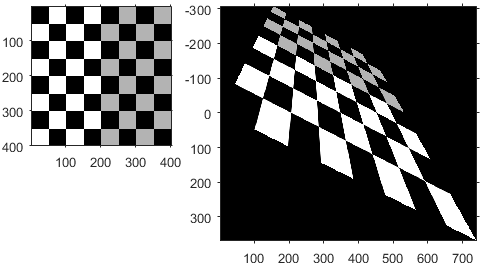`$\left[\begin{array}{ccc}1& 0& E\\ 0& 1& F\\ 0& 0& 1\end{array}\right]$`

`E` and `F` influence the vanishing point.

When `E` and `F` are large, the vanishing point comes closer to the origin and thus parallel lines appear to converge more quickly.

Note that when `E` and `F` are equal to 0, the transformation becomes an affine transformation.

Projective transformations are frequently used to register images that are out of alignment. If you have two images that you would like to align, first select control point pairs using `cpselect`. Then, fit a projective transformation matrix to control point pairs using `fitgeotrans` and setting the `transformationType` to `'projective'`. This automatically creates a `projective2d` geometric transformation object. The transformation matrix is stored as a property in the `projective2d` object. The transformation can then be applied to other images using `imwarp`.

### Create Composite 2-D Affine Transformations

You can combine multiple transformations into a single matrix using matrix multiplication. The order of the matrix multiplication matters.

This example shows how to create a composite of 2-D translation and rotation transformations.

Create a checkerboard image that will undergo transformation. Also create a spatial reference object for the image.

```cb = checkerboard(4,2); cb_ref = imref2d(size(cb));```

To illustrate the spatial position of the image, create a flat background image. Overlay the checkerboard over the background, highlighting the position of the checkerboard in green.

```background = zeros(150); imshowpair(cb,cb_ref,background,imref2d(size(background)))```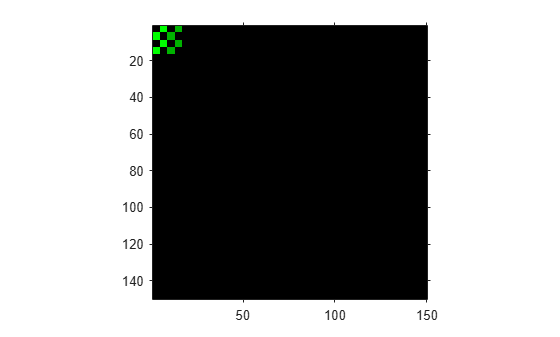Create a translation matrix, and store it as an `affine2d` geometric transformation object. This translation will shift the image horizontally by 100 pixels.

```T = [1 0 0;0 1 0;100 0 1]; tform_t = affine2d(T);```

Create a rotation matrix, and store it as an `affine2d` geometric transformation object. This translation will rotate the image 30 degrees clockwise about the origin.

```R = [cosd(30) sind(30) 0;-sind(30) cosd(30) 0;0 0 1]; tform_r = affine2d(R);```

Translation Followed by Rotation

Perform translation first and rotation second. In the multiplication of the transformation matrices, the translation matrix `T` is on the left, and the rotation matrix `R` is on the right.

```TR = T*R; tform_tr = affine2d(TR); [out,out_ref] = imwarp(cb,cb_ref,tform_tr); imshowpair(out,out_ref,background,imref2d(size(background)))```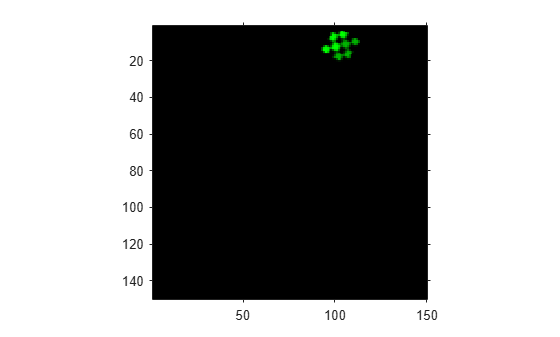Rotation Followed by Translation

Reverse the order of the transformations: perform rotation first and translation second. In the multiplication of the transformation matrices, the rotation matrix `R` is on the left, and the translation matrix `T` is on the right.

```RT = R*T; tform_rt = affine2d(RT); [out,out_ref] = imwarp(cb,cb_ref,tform_rt); imshowpair(out,out_ref,background,imref2d(size(background)))```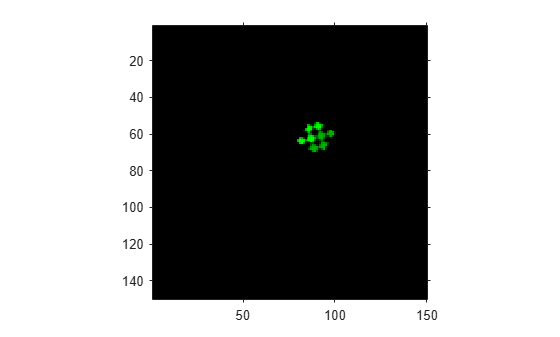Notice how the spatial position of the transformed image is different than when translation was followed by rotation.

### 3-D Affine Transformations

The following table lists the 3-D affine transformations with the transformation matrix used to define them. Note that in the 3-D case, there are multiple matrices, depending on how you want to rotate or shear the image. The last column must contain [0 0 0 1].

Use any combination of 3-D transformation matrices to create an `affine3d` geometric transformation object. Use combinations of 3-D translation and rotation matrices to create a `rigid3d` geometric transformation object.

3-D Affine TransformationTransformation Matrix
Translation

`$\left[\begin{array}{cccc}1& 0& 0& 0\\ 0& 1& 0& 0\\ 0& 0& 1& 0\\ {t}_{x}& {t}_{y}& {t}_{z}& 1\end{array}\right]$`

Scale

`$\left[\begin{array}{cccc}{s}_{x}& 0& 0& 0\\ 0& {s}_{y}& 0& 0\\ 0& 0& {s}_{z}& 0\\ 0& 0& 0& 1\end{array}\right]$`

Shearx,y shear:

`$\begin{array}{l}x\text{'}=x+az\\ y\text{'}=y+bz\\ z\text{'}=z\end{array}$`

`$\left[\begin{array}{cccc}1& 0& 0& 0\\ 0& 1& 0& 0\\ a& b& 1& 0\\ 0& 0& 0& 1\end{array}\right]$`

x,z shear:

`$\begin{array}{l}x\text{'}=x+ay\\ y\text{'}=y\\ z\text{'}=z+cy\end{array}$`

`$\left[\begin{array}{cccc}1& 0& 0& 0\\ a& 1& c& 0\\ 0& 0& 1& 0\\ 0& 0& 0& 1\end{array}\right]$`

y, z shear:

`$\begin{array}{l}x\text{'}=x\\ y\text{'}=y+bx\\ z\text{'}=z+cx\end{array}$`

`$\left[\begin{array}{cccc}1& b& c& 0\\ 0& 1& 0& 0\\ 0& 0& 1& 0\\ 0& 0& 0& 1\end{array}\right]$`

`$\left[\begin{array}{cccc}1& 0& 0& 0\\ 0& \mathrm{cos}\left(a\right)& \mathrm{sin}\left(a\right)& 0\\ 0& -\mathrm{sin}\left(a\right)& \mathrm{cos}\left(a\right)& 0\\ 0& 0& 0& 1\end{array}\right]$`

`$\left[\begin{array}{cccc}\mathrm{cos}\left(a\right)& 0& -\mathrm{sin}\left(a\right)& 0\\ 0& 1& 0& 0\\ \mathrm{sin}\left(a\right)& 0& \mathrm{cos}\left(a\right)& 0\\ 0& 0& 0& 1\end{array}\right]$`

`$\left[\begin{array}{cccc}\mathrm{cos}\left(a\right)& \mathrm{sin}\left(a\right)& 0& 0\\ -sin\left(a\right)& \mathrm{cos}\left(a\right)& 0& 0\\ 0& 0& 1& 0\\ 0& 0& 0& 1\end{array}\right]$`

For N-D affine transformations, the last column must contain ```[zeros(N,1); 1]```. `imwarp` does not support transformations of more than three dimensions.